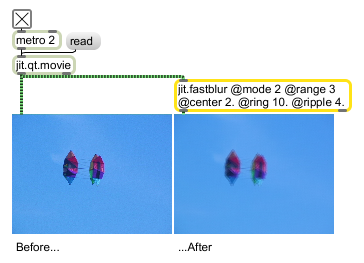# jit.fastblur

Optimized blur/sharpen

## Description

The jit.fastblur object performs several special-case convolution blur/sharpen operations on incoming matrices. Although it is less flexible than the jit.convolve object, the jit.fastblur object will be quicker and easier to set up for the equivalent convolution, in most cases.

## Matrix Operator

matrix inputs:1, matrix outputs:1

## Attributes

Name Type g/s Description
center float The mathematical weighting of the center pixel when performing convolution (default = 1.)
mode int The kernel shape (default = 0 (row))
0 = row
1 = column
2 = cross
3 = diagonal
4 = square
range int The number of pixels from the center pixel of the convolution kernel to its edge (default = 1)
ring float The mathematical weighting of cells adjoining the center pixel when performing convolution (default = 1.)
ripple float The scaling applied to outer cells when performing convolution (default = 1.) The second ring of pixels is scaled by this factor in relation to the ring attribute when performing convolution, while the third ring is scaled in relation to the second.

## Examples